# 自学Python2.8-条件(if、if...else）

### 1.if 判断语句

if语句是用来进行判断的，其使用格式如下：

 if 要判断的条件:
条件成立时，要做的事情
• 当“判断条件”成立（True）时，才执行语句；反之，则不执行。
• 执行语句可以为多行，以缩进来区分表示同一范围。
• 在 Python 中，非零值表示 True；None 和 0 表示 False。
price = input("请问这苹果多钱一斤：")
price_num = int(price)
if price_num> 5:
print('纳尼，居然', price)
print('简直太贵了！')
print("货比三家，再转转。")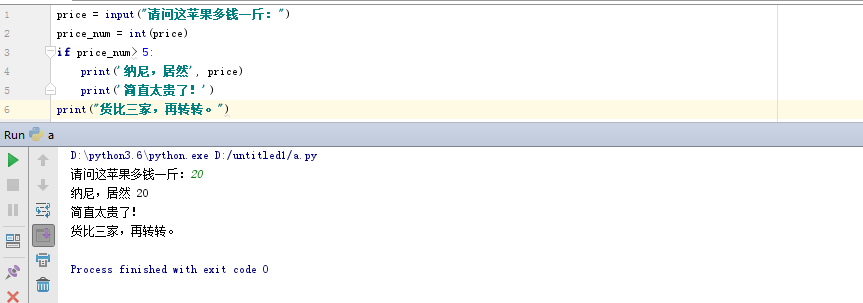### 2. if…else 语句   if ....elif…else

单分支判断语句
if 条件：
内容1
内容2
else：
内容3
内容4

多分支判断语句
if 条件：
内容1
内容2
elif 条件
内容3
elif 条件
内容4
else:
内容5

• elif 是 else if 的缩写，允许我们检查多个表达式。
• 如果 if 的条件为 False，则检查下一个 elif 的状态，依次进行。。。倘若所有条件都为 False，则执行 else 中的语句。
•  if 和 else 只能有一个，但 elif 可以有多个，if … elif … else 中只有一个语句块可以根据条件来执行。

python中的比较运算符如下表：

== 检查两个操作数的值是否相等，如果是则条件变为真。 如a=3,b=3则（a == b) 为 true.
!= 检查两个操作数的值是否相等，如果值不相等，则条件变为真。 如a=1,b=3则(a != b) 为 true.
<> 检查两个操作数的值是否相等，如果值不相等，则条件变为真。 如a=1,b=3则(a <> b) 为 true。这个类似于 != 运算符
> 检查左操作数的值是否大于右操作数的值，如果是，则条件成立。 如a=7,b=3则(a > b) 为 true.
< 检查左操作数的值是否小于右操作数的值，如果是，则条件成立。 如a=7,b=3则(a < b) 为 false.
>= 检查左操作数的值是否大于或等于右操作数的值，如果是，则条件成立。 如a=3,b=3则(a >= b) 为 true.
<= 检查左操作数的值是否小于或等于右操作数的值，如果是，则条件成立。 如a=3,b=3则(a <= b) 为 true.

python中的逻辑运算符如下表：

and x and y 布尔"与" - 如果 x 为 False，x and y 返回 False，否则它返回 y 的计算值。 (a and b) 返回 20。
or x or y 布尔"或" - 如果 x 是 True，它返回 True，否则它返回 y 的计算值。 (a or b) 返回 10。
not not x 布尔"非" - 如果 x 为 True，返回 False 。如果 x 为 False，它返回 True。 not(a and b) 返回 False

python中的赋值运算符如下表：

= 简单的赋值运算符 c = a + b 将 a + b 的运算结果赋值为 c
+= 加法赋值运算符 c += a 等效于 c = c + a
-= 减法赋值运算符 c -= a 等效于 c = c - a
*= 乘法赋值运算符 c *= a 等效于 c = c * a
/= 除法赋值运算符 c /= a 等效于 c = c / a
%= 取模赋值运算符 c %= a 等效于 c = c % a
**= 幂赋值运算符 c **= a 等效于 c = c ** a
//= 取整除赋值运算符 c //= a 等效于 c = c // a

price = input("请问这苹果多钱一斤：")
price_num = int(price)
if price_num> 5:
print("纳尼，居然", price)
print("简直太贵了！")
print("货比三家，再转转。")
else:
print(price,"还算地道")
print("给我来二斤")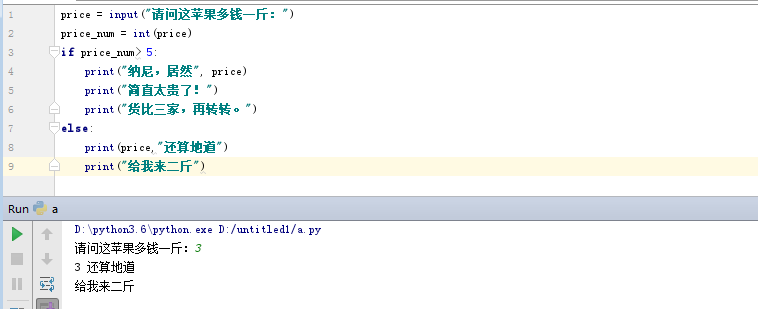score = input("请输入您的分数：")
score_num = int(score)
if score_num>=90 and score_num<=100:
print ("本次考试,等级为A")
elif score_num>=80 and score_num<90:
print ("本次考试,等级为B")
elif score_num>=70 and score_num<80:
print ("本次考试,等级为C")
elif score_num>=60 and score_num<70:
print ("本次考试,等级为D")
elif score_num>=0 and score_num<60:
print ("本次考试,等级为E")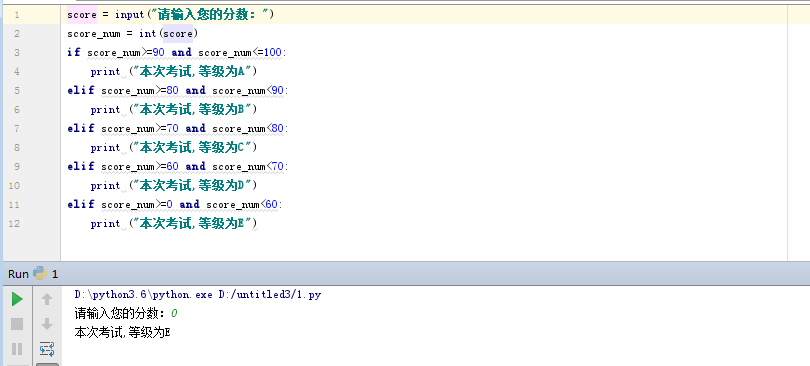name = input("please input username:")
print("yes")
print("yes")
else:
print("no")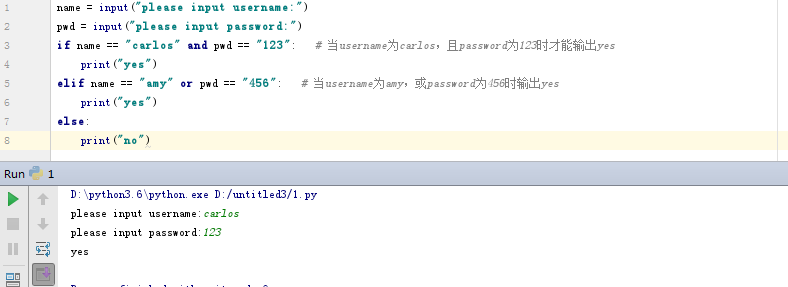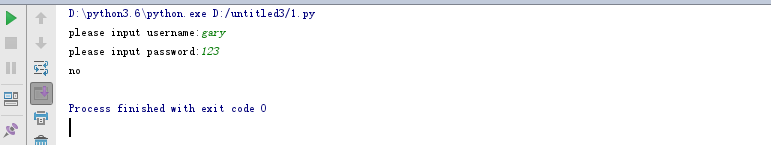color = input("你白么：")
money = input("请输入你的财产：")
beautiful = input("你美么：")
if color == "白" and money >= "100000" and beautiful == "美":
print("哇...白富美")
else:
print("滚蛋")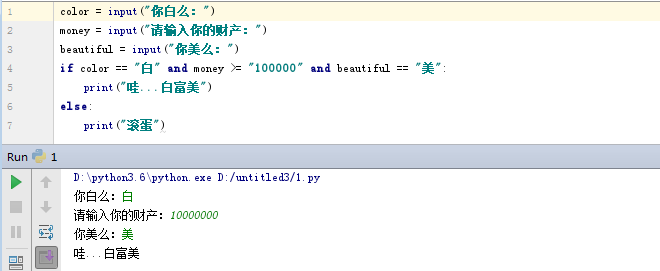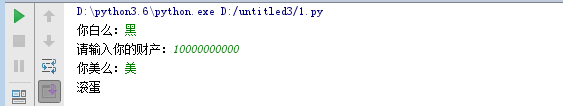a = input("请输入一个整数：")
a_num=int(a)
if a_num>0 and a_num<=50:
print("a在0到50之间")
a = input("请输入一个整数：")
if not(a_num<0 and a_num>=50):
print("a在0到50之间")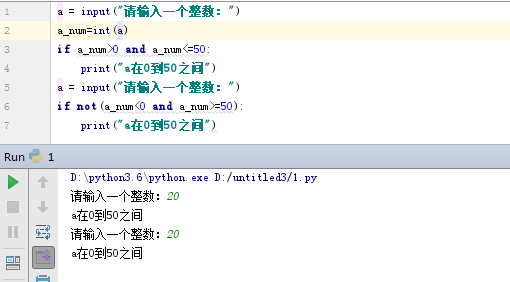### 3. 嵌套语句

    if 条件1:

满足条件1 做的事情1
满足条件1 做的事情2
...(省略)...

if 条件2:
满足条件2 做的事情1
满足条件2 做的事情2
...(省略)...

chePiao = 1     # 用1代表有车票，0代表没有车票
daoLenght = 9   # 刀子的长度，单位为cm
if chePiao == 1:
print ("有车票，可以进站")
if daoLenght < 10:
print ("通过安检")
print ("终于可以见到Ta了，美滋滋")
else:
print("没有通过安检")
print("刀子的长度超过规定，等待警察处理..")
else:
print ("没有车票，不能进站")
print ("亲爱的，那就下次见了，一票难求啊~~)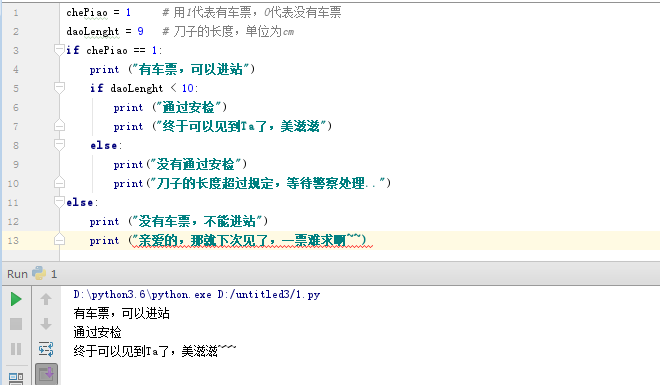posted on 2018-10-16 07:12  CARLOS_CHIANG  阅读(...)  评论(...编辑  收藏